0
154

Question 1: A boy starts from home, which faces south. He walks a distance of 10 m, turns right and walks 10 m. Then he turnsright and walks 10 m, then turns left and walks 10 m and walks back 5 m. Whatis the distance between the homeandthe boy ?

a) 35m

b) 45m

c) 15m

d) 20 m

Solution:

Let the house be located at (0,0).
Then the stops along the journey made by the boy are:
(0,-10) –> (-10,-10) –> (-10,0) –> (-20,0) –> (-15,0)
Hence, the distance between house aand the boy is 15 m

Question 2: Sachin walked 10 kms to north, turnedleft and walked 5 kms. Again he turned south and walked 4 kms.Finally, again he turned left and walked 5kms .In which direction is sachin from the starting point?

a) East

b) West

c) North

d) South

Solution:

Let the starting point be (0,0)
The end point is (0,6)
Hence, it is to the north of the starting point.

Question 3: Ram started walking towards East. After 1 km, he turned South and walked 5 km. Again he turned East and walked 2 km. Finally, he turns to the North and walked 9 km. How faris he from the starting point ?

a) 5 km

b) 7 km

c) 3 km

d) 4 km

Solution:

Let starting point be (0,0) The respective points on the journey up ahead are: (1,0), (1,-5), (3,-5), (3,4).
The journey stops at (3,4) finally.
Distance between starting and ending points = 5 km.

Question 4: Going 60 metresto the South of his house, Kiran turns left and goes another 20 metres then turning to the North, he goes 40 metres and then starts walking to his house. In which direction is his house. In which direction is his house from there ?

a) East

b) North West

c) North

d) South East

Solution:

Let the house be located at (0,0)
Just before starting journey towards house again, Kiran is at point (20, -20)
This is in the fourth quadrant.
Hence, from (20, -20) the house at (0,0) lies in the north west direction.

Question 5: The door of Priya’s house faces East. From the back side of the house, she walks straight 50 meters, then turns to the right and walks 50 meters again. Finally, she turns towards the left and stops afler walking 25 meters. Now Priya is facing which direction ?

a) North

b) West

c) East

d) South

Solution:

The back door of Priya’s home will lead her to West. She walks 50 meters West.
Then she turns right and hence, walks 50 meters North.
Then, again when she turns left, she is facing west again and thus ends up facing West when she stops walking after 25 meters

Question 6: Ram travelled 6 ft towards West, he turned left and walked 8 ft, then turned left and walked 4 ft, then turned left and walked 8 ft again. How far is he now from the starting point ?

a) 8 ft

b) 6 ft

c) 4 ft

d) 2 ft

Solution: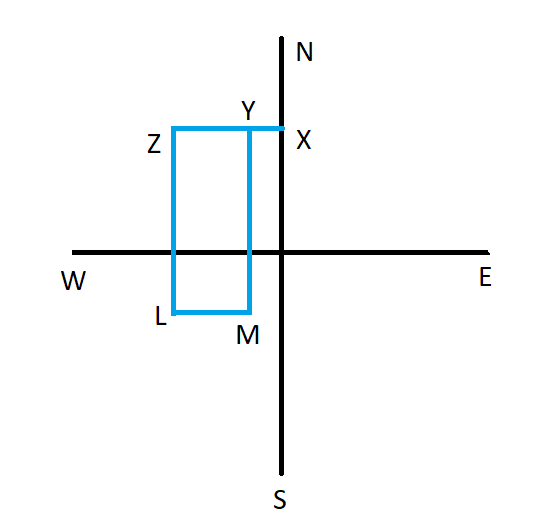X-Z-L-M-Y is the path followed by Ram. Hence, distance of end point from starting point = XY = 2 ft

Question 7: If an electric train runs in the direction from North to South with a speed of 150 km/hr covering 2000 km, then in which direction will the smoke of its engine go ?

a) $N \rightarrow S$

b) $S \rightarrow N$

c) $E \rightarrow W$

d) No direction

Solution:

An electric train does not emit smoke. Therefore, no smoke will be going in any of the direction.

Question 8: From point A, Ravi walks 5 km North-West to point B, from point B he walks 10 km South to point ‘C’. From point Che moves 5 km North – East to point D. From point D he was back to point A. If Ravi always walked in a straight line what figure has he traced ?

a) Trapezium.

b) Rhombus

c) Kite

d) Parallelogram

Solution: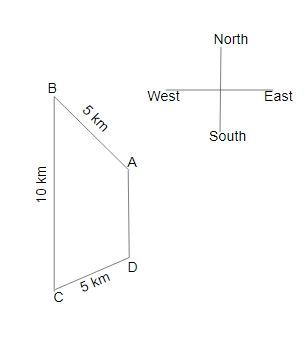From the diagram, Ravi traced the Trapezium figure.

Question 9: Ram started from his house and travelled 3 km towards South. Then turned left and travelled 4 km. Then again he turnedright andtravelled 3 km. From there, he turnedleft and travelled 4 km. At what distance is he now from his house ?

a) 15 km

b) 5 km

c) 10 km

d) 14 km

Solution: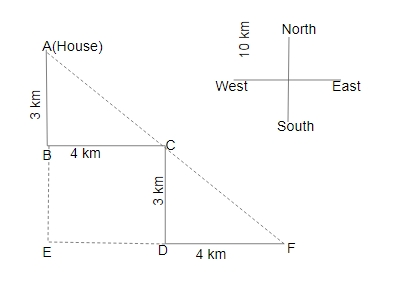From the figure,

AE = 3 + 3 = 6 km

EF = 4 + 4 = 8 km

In $\triangle$ AEF,

$(AF)^2 = (AE)^2 + (EF)^2$

$(AF)^2 = (6)^2 + (8)^2$

$(AF)^2 = 36 + 64$

$(AF)^2 = 100$

AF = 10 km

Distance = 10 km

Question 10: Hospital is 12 km towards east of Rupin’s house. His school is 5 km towards south of Hospital. What is the shortest distance between Rupin’s house and school?

a) 16 km

b) 17 km

c) 12 km

d) 13 km

Solution: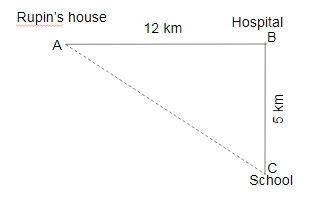From the Pythagoras,

$(AC)^2 = (AB)^2 + (BC)^2$

$(AC)^2 = (12)^2 + (5)^2$

$(AC)^2 = 169$

AC = 13 km

Shortest distance = 13 km.

Question 11: Two women start walking from the same point. Woman A walks 3 m West. Then she turns to her left and walks 10 m, then she turns to her left again and walks 9 m. At the same time, B has walked 6 m East, then she turns to her right and walks 2 m. Where is B now with respect to the position of A?

a) B is 12 m to the North of A

b) B is 8 m to the North of A

c) B is 8 m to the South of A

d) B is 12 m to the South of A

Solution: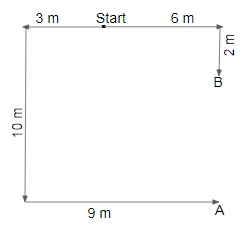Distance A to B = 10 – 2 = 8 m

$\therefore$ B is 8 m to the north of A.

Question 12: A man walks 7 km North, then turns West and walks 3 km, then turns South and walks 7 km, then turns to his right and walks 5 kms. Where is he now with reference to his starting position?

a) 2 km to the West of the starting point.

b) 8 km to the East of the starting point.

c) 8 km to the West of the starting point.

d) 2 km to the East of the starting point

Solution: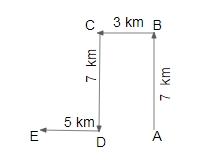Distance between E to A = 5 + 3 = 8 km

$\therefore$ Man is 8 km to the west of the starting point.

Question 13: A is standing 6 m to the East of B. A walks 9 m South, then turns to his right and walks 7 m. At the same time, B has walked 3 m West, then he turned South and
walked 9 m, then he turned to his left and walked 5 m. Where is B now with respect to the position of A?

a) B is 12 m to the East of A

b) B is 3 m to the West of A

c) B is 3 m to the East of A

d) B is 12 m to the West of A

Solution: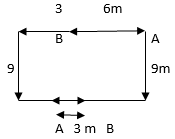$\therefore$ B is 3 m to the east of A.

Question 14: A girl walks 3 km East starting from her home. She then turns South and walks 2 km, then she turns West and walks 7 km, then she turns to her right and walks 2 km. Where is she now from her starting position?

a) 10 km to the West from her home.

b) 4 km to the East from her home

c) 10 km to the East from her home

d) 4 km to the West from her home.

Solution: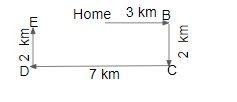Distance E to home = 7 – 3 = 4 km

Hence, she is4 km to the West from her home.

Question 15: Two A and B girls start from the same point. A walks 9 m North, then turns to her right and walks 5 m. At the same time, B Walks 11 m East, the turns to his left and walks 9 m. Where is B now with respect to the position of A?

a) B is 6 m to the East of A

b) B is 16 m to the East of A

c) B is 6 m to the West of A

d) B is 16 m to the West of A

Solution: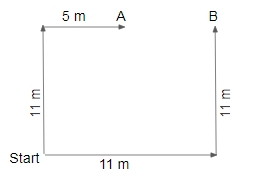Distance between A to B = 11 – 5 = 6 m

B is 6 m to the East of A.

Question 16: A wolf is chasing a deer. The wolf runs 5 km East, then turns South and runs 9 km, then turns West and runs 5 km, then it turns to its right and runs 3 km. Where is it now with reference to its starting position?

a) 12 km South from the starting position

b) 6 km North from the starting position

c) 6 km South from the starting position

d) 12 km North from the starting position

Solution: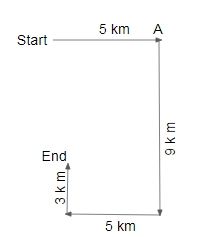Distance between starting and end point = 9 – 3 = 6 km

B is 6 m to the East of A.

$\therefore$ The correct answer is option C.

Question 17: Two people A and B start walking from the same point. A walks 15 m East, then turns south and walks 5 m. At the same time, B walks 5 m South, then he turns to his left and walks 25 m. Where is B now with respect to the position of A?

a) B is 35 m to the East of A

b) B is 10 m to the East of A

c) B is 10 m to the West of A

d) B is 35 m to the West of A

Solution: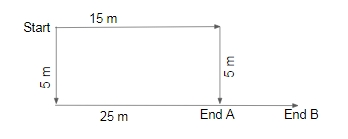Distance between end point A to B = 25 – 15 = 10 m

So, B is 10 m to the East of A.

$\therefore$ The correct answer is option B.

Question 18: A fishing boat sails 5 km South in still waters, then turns East and sails 8 km, then turns North and sails 5 km, then turns to its right and sails 10 km. Where is the boat now with reference to its starting position?

a) 18 km to the East of the starting position.

b) 2 km to the West of the starting position.

c) 18 km to the West of the starting position.

d) 2 km to the East of the starting position.

Solution: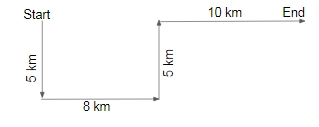Distance between Starting and end point = 8 + 10 = 18 km.

So, 18 km to the East of the starting position.
$\therefore$ The correct answer is option A.

Question 19: Two children A and B are playing. B is standing 5 m to the North of A. B then walks 10 m East, then turns to his right and walks 8 m. At the same time, A has walked 2 m to the west, then has turned to his left and walked 3 m. Where is B now with respect to the position of A?

a) B is 8 m to the East of A

b) B is 12 m to the West of A

c) B is 12 m to the East of A

d) B is 8 m to the West of A

Solution: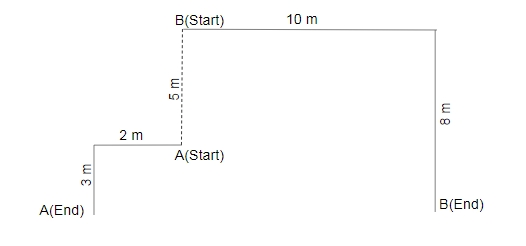Distance between end points = 10 + 2 = 12 km
So, B is the 12 m to the east of A.
$\therefore$ The correct answer is option C.

Question 20: The marathon route goes 6 km South from the starting point. It then turns West and goes 6 kms, then turns North and goes 6 km, finally it turns left and goes 2 km to reach the end point. Where is the end point with reference to the start point?

a) 4 km to the West of the starting point.

b) 8 km to the West of the starting point

c) 8 km to the East of the starting point.

d) 4 km to the East of the starting point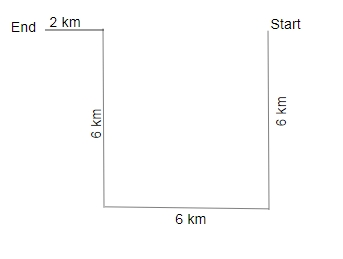$\therefore$ The correct answer is option B.